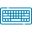# Fourier Series Calculator## Table of Content

Feedback

This Fourier series calculator is designed to find the Fourier series expansion for given functions with a complete solution. In this way, you can get any variable function's Fourier coefficients.

## What Is Fourier Series?

“Fourier series is a term that is used to indicate the expansion of periodic functions as an infinite sum of simple sine and cosine functions”.

Fourier series allows any arbitrary periodic signal with the combination of sine and cosine.

## How Fourier Series Calculator Function?

To synthesize the harmonic content of your signals or solve new differential equations
with our incredible Fourier coefficients calculator. It operates well by having the following values:

#### Required Data:

• Select the function variable and enter its value

• Also, insert the lower and upper limit

#### Result Summary:

• Fourier Series: Step-by-step Fourier series for the given functions with formula

• Coefficient Insights: Obtain the Fourier coefficients a(0), a(n), and b(n)

• Harmonic Components: Get the individual harmonic components contributing to the Fourier series expansion.

## Fourier Series Formula:

For periodic functions, the Fourier series is concluded from the Laurent expansions. It defines the orthogonality relation between the sin and cosine functions. The formula for this series of the function f(x) in the interval [-L, L], i.e. -L ≤ x ≤ L is given by:

$$f(x)=\frac{1}{2}a_{0}+\sum_{n=1}^{\infty}a_{n}cos\;nx+\sum_{n=1}^{\infty}b_{n}sin\;nx$$

Where:

$$a_0 = \frac{1}{\pi} \int_{- \pi}^{\pi} f(x) dx$$
$$a_n = \frac{1}{\pi} \int_{-\pi}^{\pi} f(x)cos\;nx\;dx$$
$$b_n = \frac{1}{\pi} \int_{-\pi}^{\pi}f(x)sin\;nx\;dx$$
$$n = 1, 2, 3$$

By determining a function as a sum of sines and cosines many problems become easy to analyze. Therefore, a Fourier series calculator is designed to help you to calculate the complex Fourier series of a given function.

## How to Calculate Fourier Series?

A Fourier series is a way to deal with functions in terms of trigonometric functions. It is prominent in partial differential equations. It is calculated by using the simple Fourier series calculator.

So look at the example below that will help you to understand their calculation process in a better way.

### Practical Example:

Calculate the Fourier series of a function f(x) = 2 - x² over an interval [-1, 1].

Given Data:

To Find: $$2 - x^{2}$$

On Interval: $$\left[- \pi,\pi\right]$$

#### Solution:

As we know the formula is considered valuable by the Fourier expansion calculator.

$$f(x)=\frac{1}{2}a_{0}+\sum_{n=1}^{\infty}a_{n}cos\;nx+\sum_{n=1}^{\infty}b_{n}sin\;nx$$

Find the values:

$$A_0,A_n \text{ and } B_n$$

$$A_0=\frac{1}{2\times \pi}\cdot \int _{- \pi}^\pi\left(2 - x^{2}\right)dx = 4 - \frac{2 \pi^{2}}{3}$$

$$A_n=\frac{1}{\pi}\cdot \int _{- \pi}^\pi\left(2 - x^{2}\right)\cos \left(\frac{n\pi x}{\pi}\right)dx = - \frac{4 \left(-1\right)^{n}}{n^{2}}$$

$$B_n=\frac{1}{\pi}\cdot \int _{- \pi}^\pi\left(2 - x^{2}\right)\sin \left(\frac{n\pi x}{\pi}\right)dx = 0$$

Put the values in the formula:

$$f\left(x\right)=4 - \frac{2 \pi^{2}}{3} +\sum _{n=1}^{\infty \:}- \frac{4 \left(-1\right)^{n}}{n^{2}}\cdot \cos \left(\frac{n\pi x}{L}\right)+\sum _{n=1}^{\infty \:}0\cdot \sin \left(\frac{n\pi x}{L}\right)$$

After Simplifying:

$$\sum_{n=1}^{\infty} - \frac{4 \left(-1\right)^{n} \cos{\left(n x \right)}}{n^{2}} - \frac{\pi^{2}}{3} + 2$$

## Reference:

Wikipedia: Fourier series, Common forms of the Fourier series, Table of common Fourier series, Table of basic properties.### Alan Walker

Studies mathematics sciences, and Technology. Tech geek and a content writer. Wikipedia addict who wants to know everything. Loves traveling, nature, reading. Math and Technology have done their part, and now it's the time for us to get benefits.